# Catch up on the latest AI articles# LONGNET: Model Capable Of Processing Text Up To 1 Billion Tokens

3 main points
✔️ present an important solution to the challenge of efficient processing of long sequences
✔️ Introducing Dilated Attention to reduce the computational complexity of Transformern
✔️ LONGNET introduces Dilated Attention, a Transformer-based Model

written by Jiayu DingShuming MaLi DongXingxing ZhangShaohan HuangWenhui WangNanning ZhengFuru Wei
(Submitted on 5 Jul 2023 (v1), last revised 19 Jul 2023 (this version, v2))

Subjects:  Computation and Language (cs.CL); Machine Learning (cs.LG)code：The images used in this article are from the paper, the introductory slides, or were created based on them.

## summary

LONGNET proposes a variation of Transformer that introduces a new architecture called Dilated Attention, a technology that has become a LONGNET innovation.

By LONGNET.

- Solved the problem of the old Transformer's processing power requirements spiking as sequence lengths increased.
- Better handling of both short and long sequences
- Longer contexts were found to improve the performance of the language model.

## Benefits of being able to process 1 billion tokens

One billion tokens is equivalent to about 250,000 times as many tokens as GPT-4. No model has ever been able to handle this many tokens. It was therefore difficult to include the entire book or to input the entire web into the model.

The authors propose a way to scale sequence length linearly with LONGNET, and mention the possibility of including entire web datasets in the future. The authors also mention that the advent of LONGNET could be a paradigm shift in context learning with many real-world examples, as it allows for very long context learning.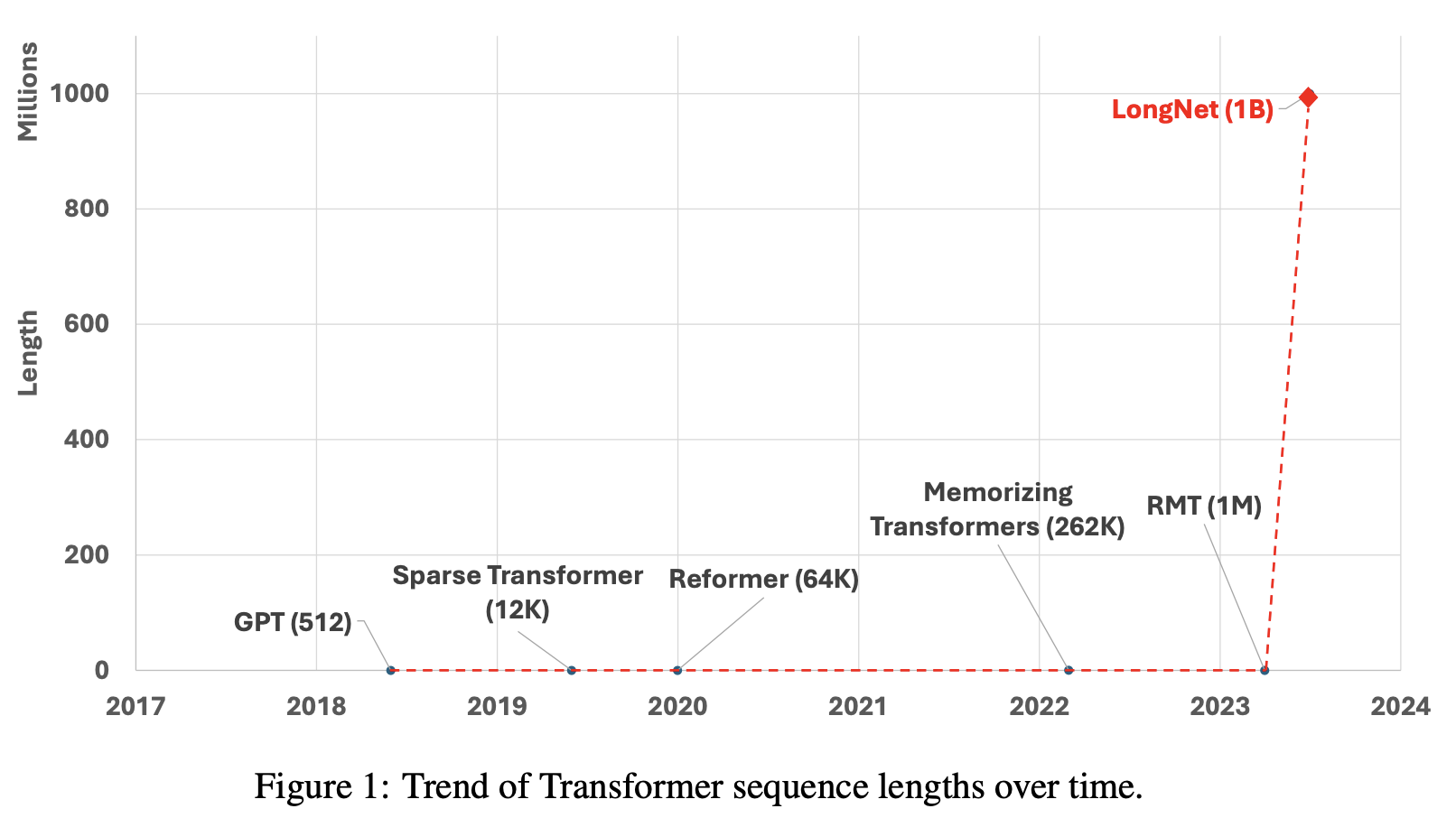## Background of the birth of LONGNET

With the advent of LONGNET and Dilated Attention, a solution to the two problems of "increasing sequence length" and "reducing the computational complexity of the transformer" has been proposed.

Although it was generally known that the benefits gained by increasing sequence length are significant, Transformer's computational complexity is a quadratic function of sequence length, and the required processing power increases dramatically. For this reason. To reduce the computational complexity of the Transformer, LONGNET introduces a new component called Dilated Attention.

## Benefits associated with increased sequence length

Sequence length is a fundamental component of neural networks and it is generally considered desirable to have an unlimitedly large sequence length. In addition, there are three advantages to increasing sequence length.

- The model can take in a wider range of context, allowing it to more accurately predict the current token using distant information. This is useful, for example, for understanding spoken language from the middle of a sentence or for comprehending long documents.
- It can learn to include more complex causal relationships and inference processes in the training data. (In the paper, it seems that short dependencies are generally detrimental.)
- Can understand longer contexts and take full advantage of them to improve the output of the language model.

## Reduced Transformer computational complexity

The computational complexity of Transfomer increases quadratically with sequence length. In contrast, the Dilated Attention proposed in this paper increases linearly.

The effect is illustrated in Figure 5 of this paper, which compares the performance of vanilla and dilated attention. The length of the sequences (from 8K to 1B) is scaled gradually. In the graph below, we record the average execution time with 10 different forward propagations for each model and compare them. Both models are implemented with the FlashAttention Kernel, which saves memory and improves speed.

dilated attention shows that the latency in scaling the sequence length is nearly constant. This makes it possible to scale sequence lengths up to one billion tokens.

On the other hand, vanilla attention is quadratically computationally expensive with respect to sequence length, resulting in a rapid increase in latency with increasing length. Furthermore, vanilla attention does not have a distributed algorithm to overcome the sequence length limitation.

This result also shows the superiority of LONGNET's linear complexity and distributed algorithms.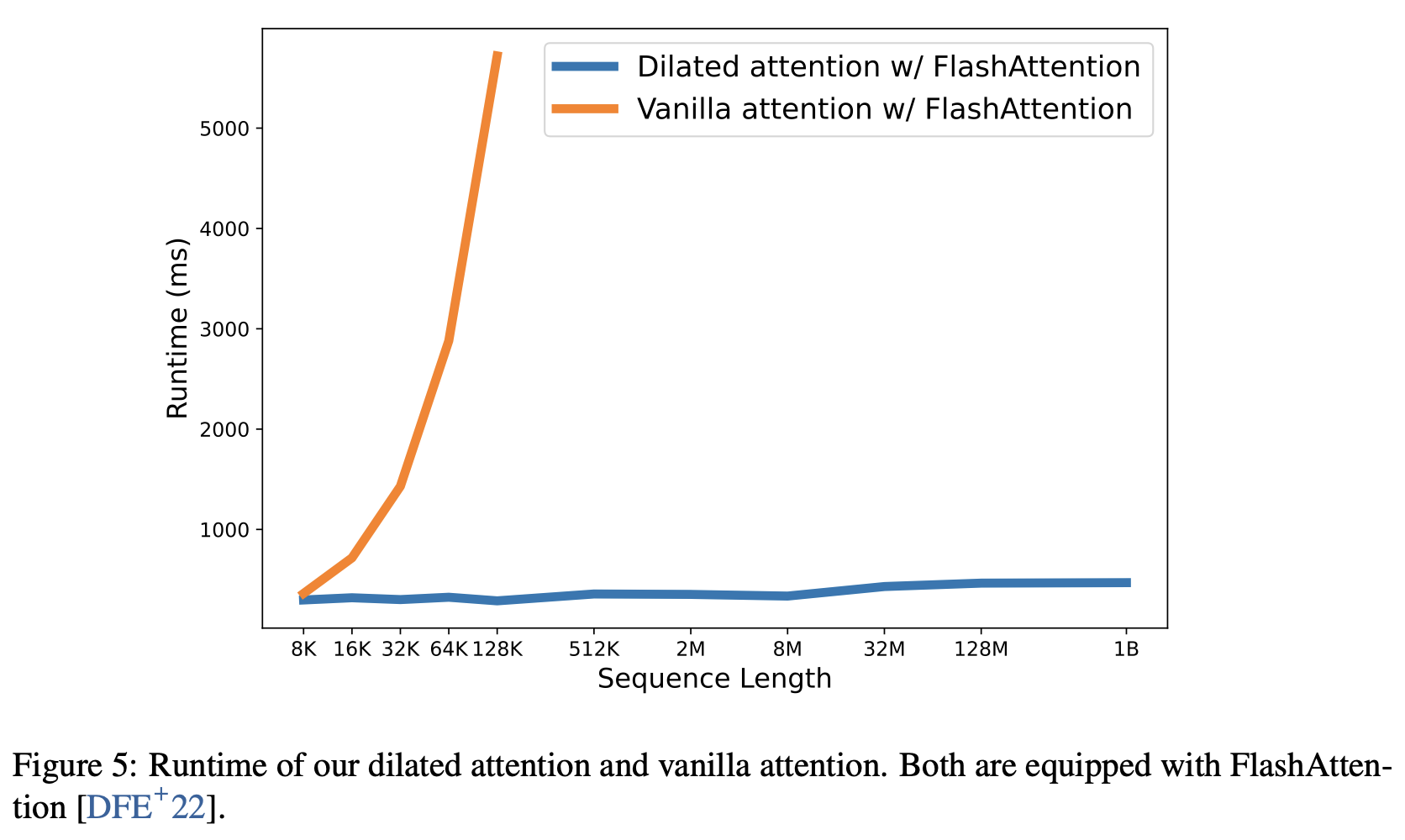## To what extent has the computational complexity improved compared to existing studies?

You may have noticed the dramatic improvement in computational complexity in the previous section. Now, let's take a look at how much the computational complexity has improved from a theoretical standpoint.

The improvement in computational complexity was achieved by adopting an architecture called Dilated Attention, a typical attempt to reduce the computational complexity of the Transformer. According to our comparison, the newly proposed Dilated Attention reduces the computational complexity of the Attention mechanism compared to the conventional Attention and Sparse Attention, as shown in the table below.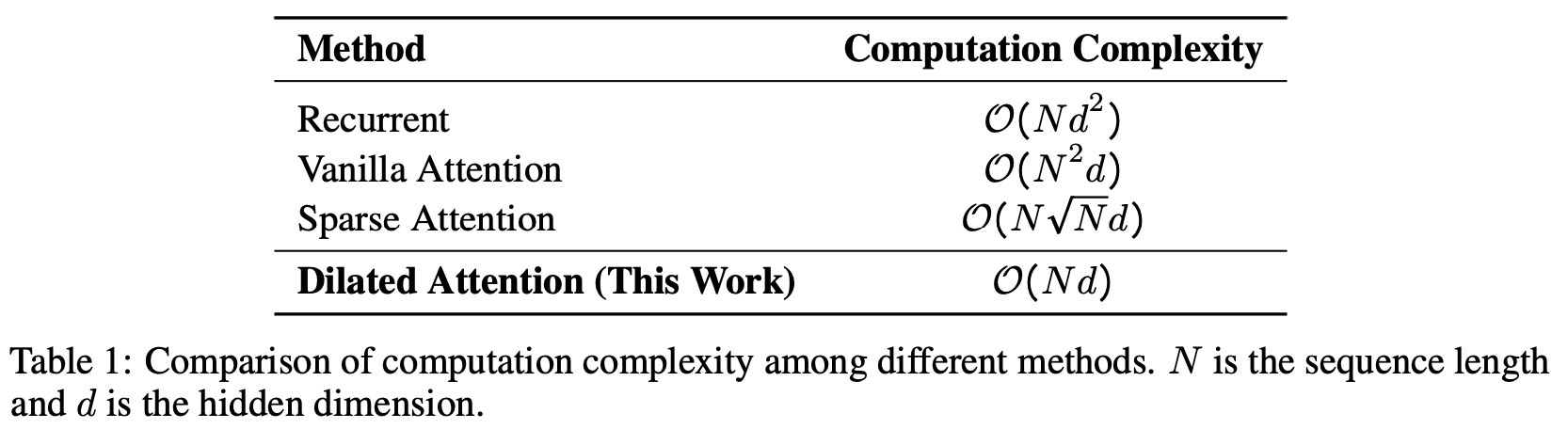In the next section, we will show how we were able to successfully reduce the computational complexity.

## Dilated Attention: Why did we improve the computational complexity?

Let's look at why Dilated Attention reduces the computational complexity from the mathematical equation.

Dilated Attention divides the input $(Q, K, V)$ into segments ${(Q, K, V)}^{\frac{N}{w}}$ with segment length $w$.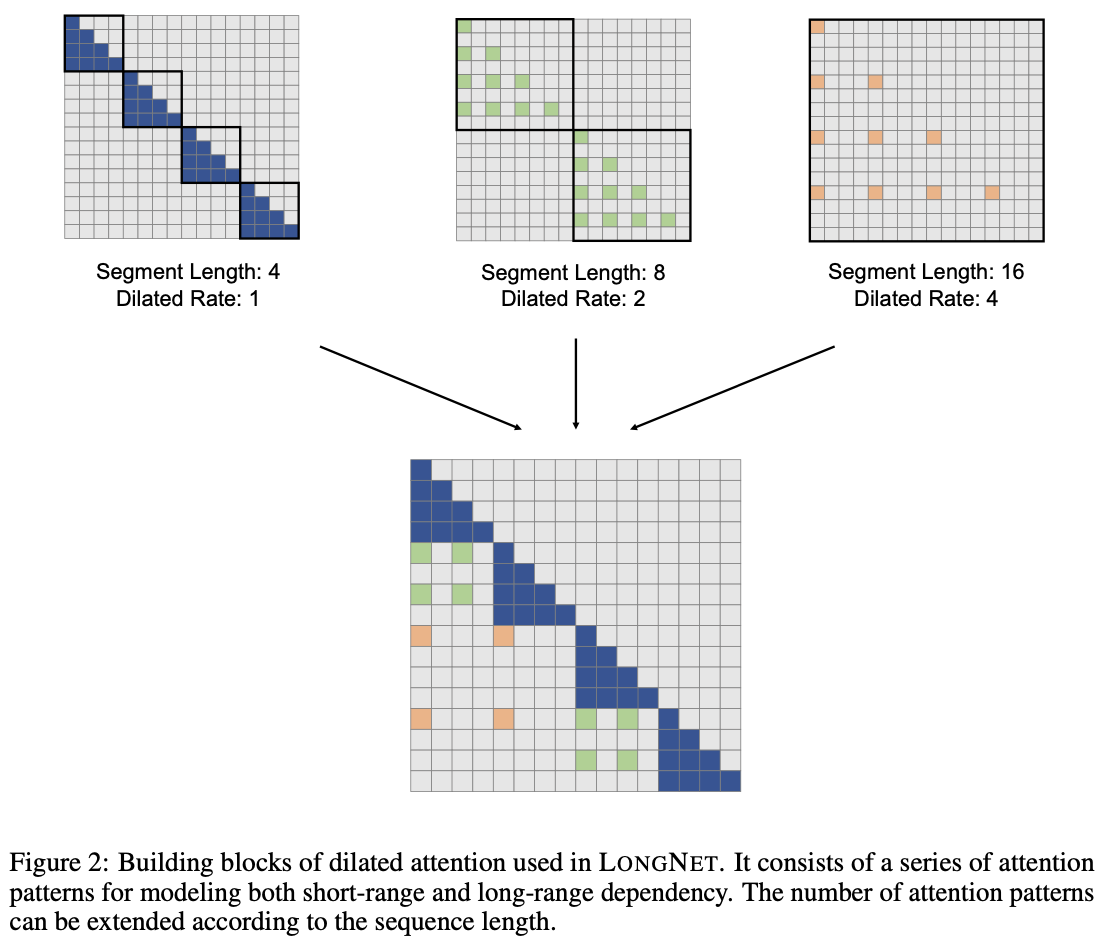Each segment is sparsified along the sequence dimension by selecting rows in the interval $r$, as shown in Figure 2. The actual formula is shown below.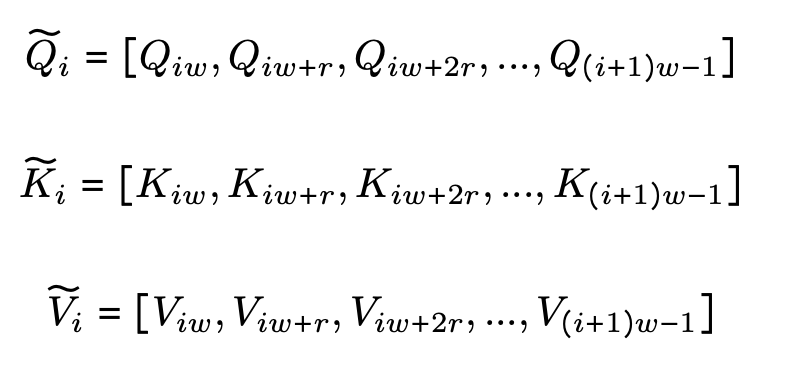This sparsified segment ${(Q, K, V)}^{\frac{N}{w}}$ is fed to Attention in parallel. After being fed, if the input sequence length is longer than the local sequence length, they are scattered, computed, and finally concatenated into the output $O$.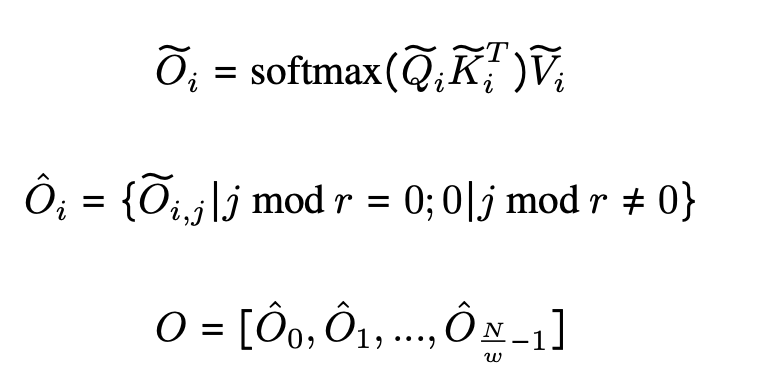Also, in the implementation, Dilated Attention can be converted to Dense Attention between collection operations on $(Q, K, V)$ input and operations on $widetilde{O_i}$ output, allowing direct use of Vanilla Attention optimizations such as Flash Attention. Attention, such as Flash Attention, can be directly exploited.

In practice, segment size $w$ trades Attention globality for efficiency. On the other hand, size $r$ reduces the computational cost by approximating it to the Dilated Attention matrix.

## Distributed Training in LONGNET

The computation order of Dilated Attention has been significantly reduced to $O(Nd)$ from $O(N^2d)$ of Vanilla Attention. However, it is not possible to scale the sequence length to the order of a million on a single GPU due to computational resource and memory constraints. Therefore, distributed training algorithms for large-scale model training have been proposed, including parallel processing of models, sequence processing, and pipelining. However, conventional methods are insufficient for LONGNET, especially when the dimension of sequences is large. Therefore, LONGNET proposes a new distributed algorithm that can be extended to multiple devices without loss of generality.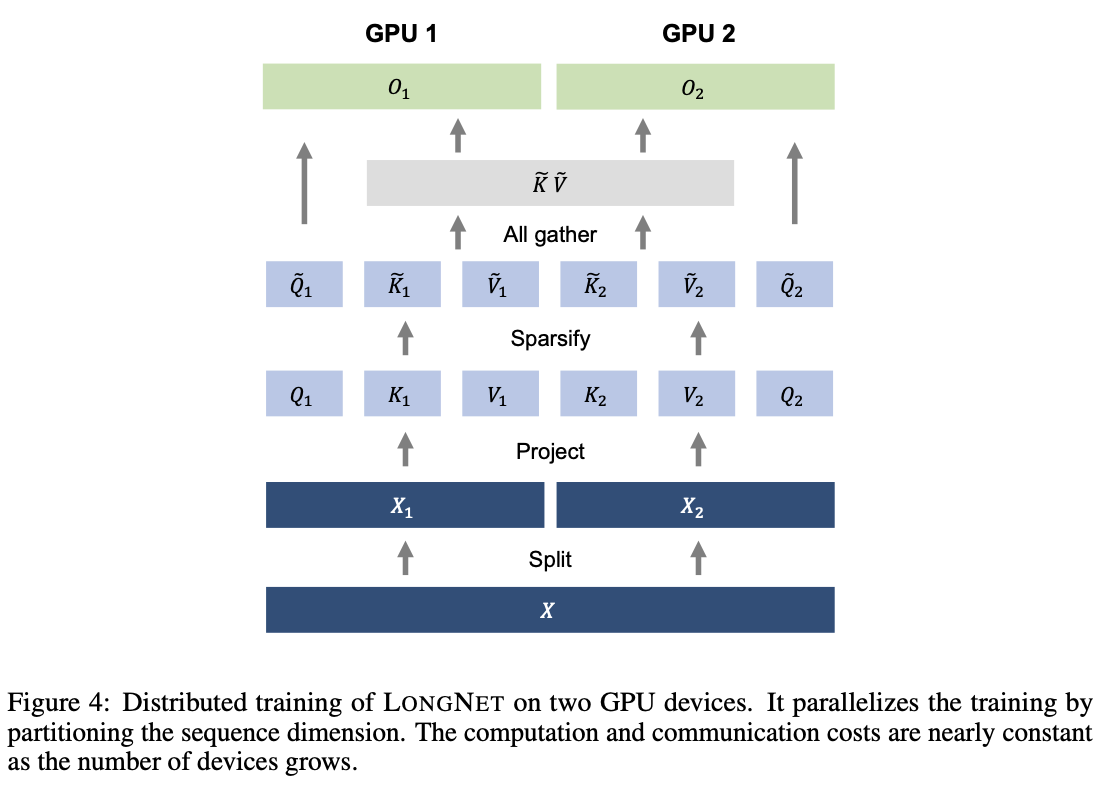### STEP1: Input Sequence Segmentation

The input sequence is divided along the sequence dimension. Each divided sequence is placed separately on a single device.

$X = [X_1 , X_2]$

The query, key, and value on the two devices are also as follows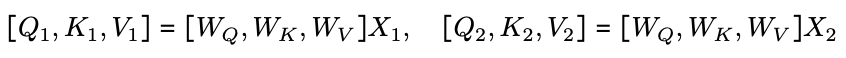### STEP2: Attention calculation

When $W_i \leq l$, that is, when the input segment length ($W_i$) is shorter than the local device sequence length ($l$), it is broken up using the calculation method introduced in Dilated Attention.

When $w_i \geq l$, keys and values are scattered on the device, so a full set operation is performed to collect keys and values before computing Attention.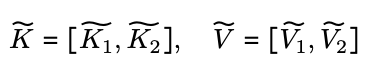At this time, unlike Vanilla Attention, neither the key nor the value size depends on the sequence length $N$, so the communication cost remains constant.

### STEP3: Calculate Cross Attention

Compute Cross Attention using local queries and global keys and values.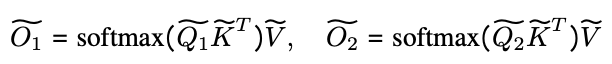### STEP4: Final output

The final Attention output is the concatenation of the outputs of the different device kanes, as shown in the equation below.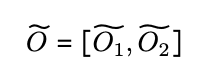## Experiments in language modeling

This paper actually implements the language model, and the architecture employed is MAGNETO [WMH+22], using XPOS [SDP+22] relative position encoding. However, it seems to replace the standard Attention with Dilated Attention.

Compare LONGNET with both Vanilla Transformer and Sparse Transformer. In scaling the sequence length of these models from 2K to 32K, it appears that the batch size is adjusted to keep the number of tokens per batch size constant. Also, due to the limitations of the authors' computing environment, they have only experimented with up to 32K tokens. Below are the perplexity results for each language model.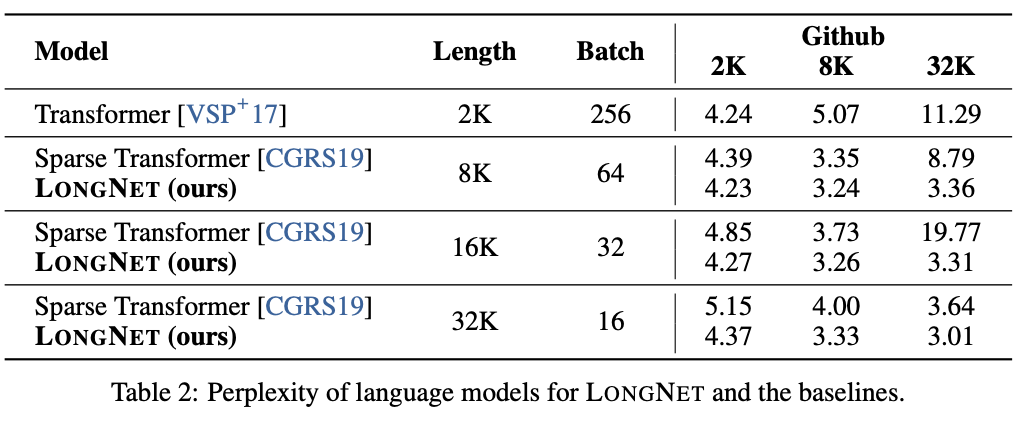The main result proves that increasing the sequence length during training yields a good language model; LONGNGET performs better than the other models in all cases, indicating its effectiveness.

Figure 6 below plots the sequence length scaling curves for both Vanilla Transformers and LONGNET. As can be seen, LONGNET is confirmed to follow the scaling law. Based on this result, it is claimed that LONGNET can scale up the context length more efficiently, and thus performs better with less computation between Vanilla Transformers and LONGNET.

## summary

The authors plan to expand the scope of LONGNET to include tasks such as multimodal large-scale language modeling, pre-training for BEiT, and genomic data modeling. This is expected to enable LONGNET to handle an even wider variety of tasks and deliver superior performance.

It also suggests that the ability to accept longer prompts may allow for more sophisticated output without the need for additional learning, such as through extensive or large numbers of examples in the prompts.

If you have any suggestions for improvement of the content of the article,